## 20141125

### Physics presentation: internal energy conservationSigh. This equation. The ubiquitous "Q = m·c·∆T" endlessly recited in chemistry, more than likely without much understanding. And by "understanding," I mean, "physics."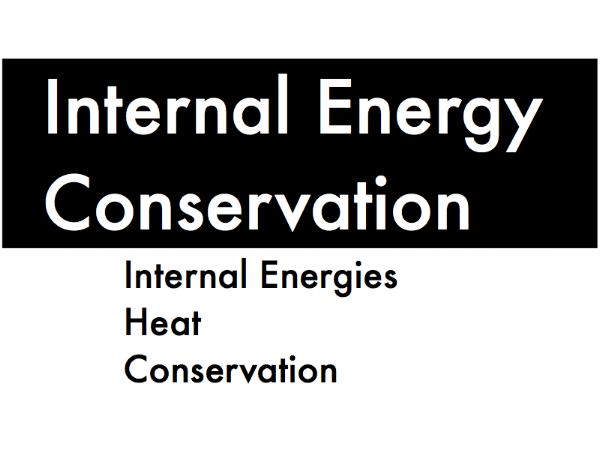Well, now that you've seen that equation, you can't unsee it, so in this presentation we'll instead emphasize the conceptual meaning of heat transfers (the "Q" on the left side of the equality), and of changes in thermal internal energy (the m·c·∆T" on the right side of the equality), and see how this equation works in the context of principles developed earlier in this course for work and mechanical energy conservation.First, introducing internal energy systems, and especially changes in thermal internal energy.Recall that mechanical energy systems such as translational kinetic energy and rotational kinetic energy are concerned with the macroscopic translational motion or rotational motion of an entire object. Thermal internal energy is also concerned with a motion of an object, more specifically the random microscopic motion of the individual atoms and molecules within an object. So for this just-cooked turkey coming out of the oven, it contains a large amount of thermal internal energy due to the very active microscopic motion of its atoms and molecules.The total thermal internal energy of an object's microscopic atomic and molecular motion depends on its mass m and its temperature T (measured in kelvins), and is expressed as joules (chemists use "calories" or "Calories" for energy--be sure to ask them why they keep using those non-Système International units). A low temperature object has very little thermal internal energy, and higher temperature it has, the more thermal internal energy it has. The intensive property of different materials (due to its atomic and molecular make-up) related to its thermal internal energy is the "specific heat capacity," in units of J/(kg·K).

(Note that while your textbook explains in detail what thermal internal energy is, it does not actually define a symbol for thermal internal energy, so for our purposes we'll use "Etherm.")

Instead of finding out how much thermal internal energy Etherm an object has, often we are more concerned with its initial-to-final change ∆Etherm, which is the final amount of thermal internal energy minus the initial amount of thermal internal energy. Notice how the common factors of mass m and specific heat capacity c are pulled out, such that the amount of change in thermal internal energy is determined by the ∆T change in temperature.Recall that mechanical energy systems such as gravitational potential energy and elastic potential energy are concerned with the storage of energy due to height of an object in a gravitational field, or due to the compression or stretching of a spring or an elastic material from its equilibrium state. Bond internal energy is concerned with the storage of energy, but stored in the spring-like bonds between the individual atoms and molecules in solids and liquids.

During vaporization (liquid turning into gas) or melting (solid turning into liquid), these bonds are broken, freeing up individual atoms or molecules. Bond internal energy increases during these processes, much like the elastic potential energy of a spring increasing as it stretches more and more, because the bonds between atoms and molecules must be stretched further apart in order to "disconnect" them from each other.

During condensation (gas turning into liquid) or freezing (liquid turning into solid) bonds begin to form between the individual atoms or molecules. Bond internal energy decreases during these processes, much like the elastic potential energy of a spring decreasing as it stretches less, because the distances between atoms and molecules must brought be closer together in order to "connect" them to each other.

(While there is a "latent heat" equation to calculate the change in the bond internal energy during phase changes (when bonds are broken or made between individual atoms and molecules), we will focus primarily on changes in thermal internal energies, so only consider situations where there are changes in temperature, but no changes in phase.)Next, heat--the "Q" in the "Q = m·c·∆T" equation.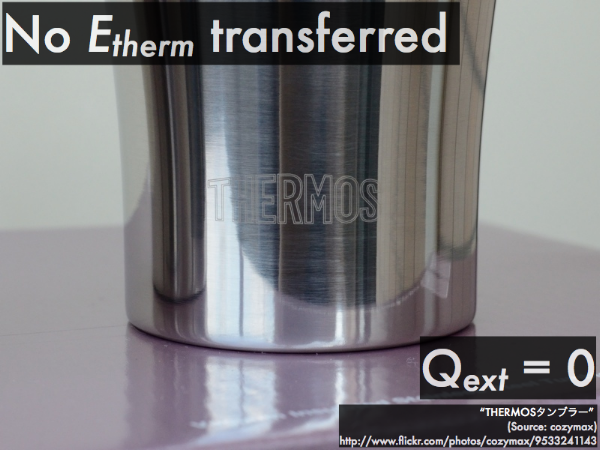Recall that work is the transfer of mechanical energy on the macroscopic level, whether put in by an external agent, or taken away because of friction/drag. Heat, then, is the transfer of internal energy on a microscopic level.

If there is no energy transferred into or out of the thermal internal energy of a system (as with the contents an extremely well-insulated Thermos® bottle), then it is effectively thermally isolated from the environment, and the heat exchanged between the system and the external environment is zero.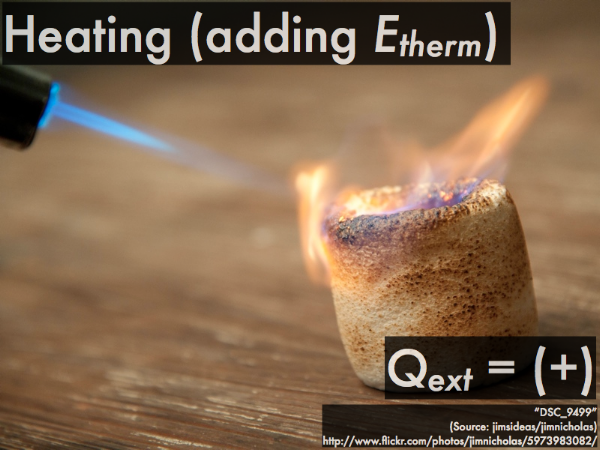If the thermal internal energy of a system increases, its temperature increases, and thus external heat from the environment is positive, being added into the system (as for this blowtorch on a marshmallow).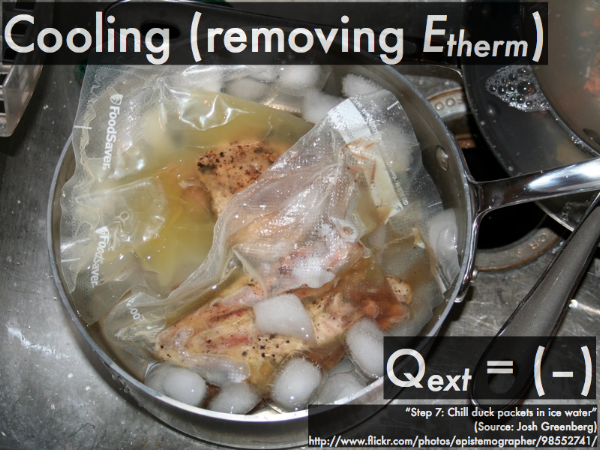If the thermal internal energy of a system decreases, its temperature decreases, and thus external heat from the environment is negative, being removed from the system (as for these sous-vide cooked portions of duck meat being chilled in an ice-water bath).The direction of heat flow is determined by the relative temperatures of two objects interacting with each other--the object at a higher temperature will "heat up" (transfer energy to) an object at a lower temperature. The two objects will stop transferring energy between each other when they attain the same final temperature (such that there is no longer a direction for heat to flow), thus reaching thermal equilibrium.

(Don't ever expect heat to spontaneously flow from a lower temperature object to a higher temperature object--unless you "do work" (expend mechanical energy) to make this happen, which is why it will cost you to run a refrigerator or air conditioner to remove heat from the low temperature contents, and dump it to the warmer environment outside. We'll focus on the "natural" direction of heat flow that occurs between two different temperature objects that thermally interact with each other.)Third, accounting for the transfers between different thermal internal energy systems, and also for the contribution to or taking from these energies by an external source of heat.This is expressed in a format in order to emphasize the parallels with the total mechanical energy conservation equation developed earlier. The transfer/balance equation here shows how the transfer of energy (as heat exchanged by external agents and/or the environment) causes corresponding changes in the thermal internal energies of all objects in our system. Any or all of these thermal energy forms on the right-hand side of the equation can increase or decrease (due to an increase or decrease in temperature), but together all of their changes must add up to the corresponding heat exchange term on the left-hand side of this equation.

When you substitute the individual ∆Etherm terms on the right-hand side of the equation with the equivalent m·c·∆T expressions, then this results in the oh-so-familiar-but-maybe-not-quite-so-meaningless-anymore "Q = m·c·∆T" equation.

Note that in the idealized case that the system is thermally isolated from both external agents and the environment, then the left-hand size of this equation would be zero. Then the individual energy terms on the right-hand side of this equation can then trade and balance amongst themselves, instead of with the outside world.

So now let's see how this transfer/balance equation can be applied to idealized situations where heat gain/loss exchanges with the outside world are negligible compared to the exchanges within a system.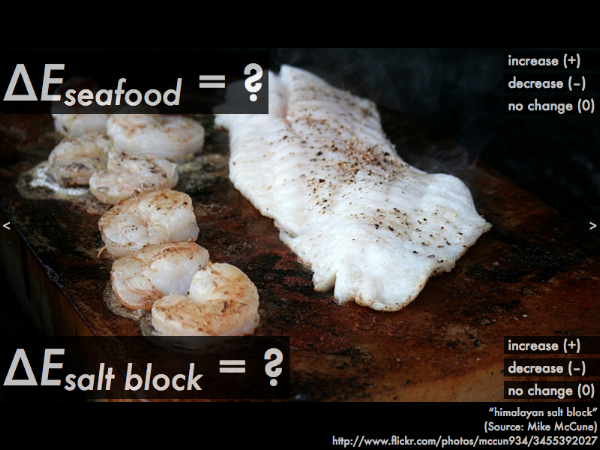Raw seafood is placed on a block of salt that has already been heated up in an oven. The energy contained in the high-temperature block of salt is then transferred to the seafood, cooking it. While it is being cooked, does the internal thermal energy of the seafood increase, decrease, or not change? Does the thermal internal energy of the salt block increase, decrease, or not change?

Assuming that the seafood and salt block system is thermally isolated from the environment (such that Qext = 0), which thermal internal energy experienced a greater amount of change: the seafood, or the block?

As the seafood cooks, its internal thermal energy increases, as the temperature of the food increases. (If you calculated the change in internal energy of the seafood, you would get a positive value, which is consistent with an increase.)

As the salt block cooks the seafood, its internal thermal energy decreases, as the temperature of the salt block decreases. (If you calculated the change in internal thermal energy of the salt block, you would get a negative value, which is consistent with a decrease.)

Since we are assuming idealized situations where heat gain/loss exchanges with the outside world are negligible compared to the exchanges within a system, then there is no heat given off or taken in from the environment, so the left-hand side of the transfer/balance equation is zero:

Qext = ∆Eseafood + ∆Esalt block,

0 = ∆Eseafood + ∆Esalt block,

then we are only left with the changes in the thermal energies of the seafood and the salt block:

0 = ∆Eseafood + ∆Esalt block,

0 = (+) + (–).

Now we can see that amount that the seafood's internal thermal energy increases (where ∆Eseafood is positive) is directly related to the amount that the salt block's internal thermal energy decreases (where ∆Esalt block is negative), in order to equal the zero on the left-hand side of the equation. So the salt block's internal thermal energy "feeds" (or is "transferred to") the seafood's internal energy during this process.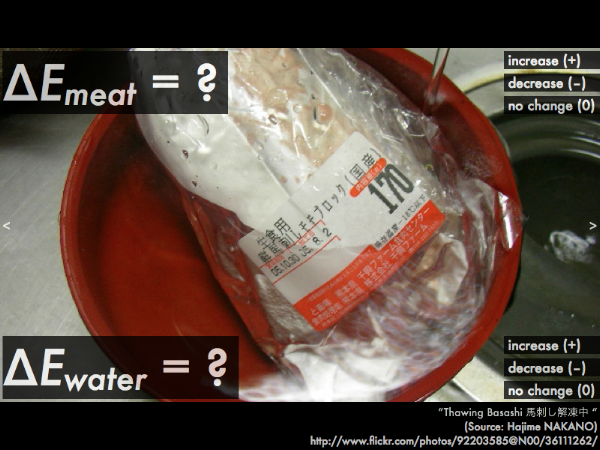Frozen meat is placed in a water bath, in order to defrost it. At the very start of this defrosting process (where the frozen meat just begins to warm up from its below-freezing temperature, and the ice crystals inside have not yet reached the melting point), does the internal thermal energy of the meat increase, decrease, or not change? Does the thermal internal energy of the water increase, decrease, or not change?

Assuming that the meat and water system is thermally isolated from the environment (such that Qext = 0), which thermal internal energy experienced a greater amount of change: the meat, or the seafood?A shot of whiskey is mixed with a pint of beer to make a boilermaker. Assuming that the whiskey and beer have approximately the same temperature before they are mixed together, does the internal thermal energy of the shot of whiskey increase, decrease, or not change? Does the thermal internal energy of the pint of beer increase, decrease, or not change?

Assuming that the whiskey and beer system is thermally isolated from the environment (such that Qext = 0), which thermal internal energy experienced a greater amount of change: the whiskey, or the beer?

(If you haven't noticed the type of vocabulary used in this presentation, we are deliberately avoiding the confusion between "hot" (in terms of high temperature, high thermal internal energy objects) and "heat" (thermal energy transferred between objects).)